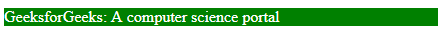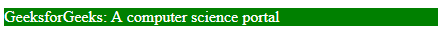Open In App

# CSS max-width Property

The max-width property in CSS is used to define the maximum width of an element. The value of the width cannot be larger than the value by max-width. If the content is larger than the max-width then it will go to the next line and if the content is smaller than the max-width then it has no effect.

Syntax:

`max-width: none | length | percentage (%) | initial | inherit;`

Property Values: All the properties are described well in the example below.

• none: It is the default value and it does not contain max-width.
• length: This property is used to set the length of max-width. The length can be set in the form of px, cm, etc.
• percentage (%): This property is used to set the max-width in the form of a percentage.
• initial: It is used to set an element’s CSS property to its default value. The initial keyword can be used for any CSS property, and on any HTML element.
• inherit: This property is used to inherit a property to an element from its parent element property value. The inherit keyword can be used for inheriting any CSS property, and on any HTML element.

Example 1: This example illustrates the use of the max-width property whose value is set to none.

## HTML

 ```<``html``>` `<``head``>``    ``<``title``>max-width property` `    ````    ``<``style``>``        ``p {``            ``max-width: none;``            ``color: white;``            ``background-color: green;``        ``}``    ````` `<``body``>` `    ``<``p``> GeeksforGeeks: A computer science portal ` `` ``

Output:Example 2: This example illustrates the use of the max-width property, in order to set the max-width length.

## HTML

 ```<``html``>` `<``head``>``    ``<``title``>max-width property` `    ````    ``<``style``>``        ``p {``            ``max-width: 110px;``            ``color: white;``            ``background-color: green;``        ``}``    ````` `<``body``>` `    ``<``p``>``      ``GeeksforGeeks: A computer science portal``    ``` `` ``

Output:Example 3: This example illustrates the use of the max-width property whose value is assigned in the form of a percentage.

## HTML

 ```<``html``>` `<``head``>``    ``<``title``>max-width property` `    ````    ``<``style``>``        ``p {``            ``max-width: 20%;``            ``color: white;``            ``background-color: green;``        ``}``    ````` `<``body``>` `    ``<``p``>``        ``GeeksforGeeks: A computer science portal``    ``` `` ``

Output:Example 4: This example illustrates the use of the max-width property, in order to set the value to its initial or default value.

## HTML

 ```<``html``>` `<``head``>``    ``<``title``>max-width property` `    ````    ``<``style``>``        ``p {``            ``max-width: initial;``            ``color: white;``            ``background-color: green;``        ``}``    ````` `<``body``>` `    ``<``p``>``        ``GeeksforGeeks: A computer science portal``    ``` `` ``

Output:Example 5: This example illustrates the use of the max-width property, with the value set to `inherit`.

## HTML

 ```<``html``>` `<``head``>``    ``<``title``>max-width property` `    ````    ``<``style``>``        ``.container {``            ``max-width: inherit;``            ``color: white;``            ``background-color: green;``        ``}``    ````` `<``body``>` `    ``<``div` `class``=``"container"``>``        ``<``p``>``            ``GeeksforGeeks: A computer science portal``        ````    ``` `` ``

Output:Supported Browsers: The browser supported by the max-width property are listed below:

• Google Chrome 1.0 and above
• Internet Explorer 7.0 and above
• Microsoft Edge 12.0 and above
• Firefox 1.0 and above
• Safari 1.0 and above
• Opera 4.0 and above